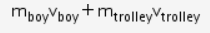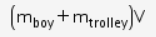# **Yes sure sir.** I want to know the meaning of the term 'common velocity'

Yes sure sir. I want to know the meaning of the term ‘common velocity’ that has been used in the following two numericals and also the concept which would be applied to solve these. 1. A boy of mass 50 kg running at 5m/s jumps on to a 20 kg trolley traveling in the same direction at 1.5 m/s. What is their common velocity? 2. A truck of mass 500 kg moving at 4m/s collides with another truck of mass 1500kg moving in the same direction at 2m/s.
What is their common velocity just after the collision if they move off together?

The term “common velocity” is used when one object collides or interacts with another object and both move together with the same velocity. Example a boy jumping on a boat, lands on it and both move with the same velocity together. Then, we will say that the boy and boat have common velocity.

In the two numericals, the concept to be used is conservation of momentum to find the common velocity of both the objects.

The initial momentum isThe final momentum will beEquating these two momenta the common velocity could be found.